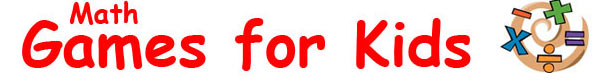Calculator Game for Kids Learn how to use a calculator with this interactive math game for kids. Find out how you can use a calculator to make life easier when solving equations. Practice with calculator functions such as all clear (AC), memory recall (MR), square root, percentage and division. Complete the calculations, get familiar with the functions of a calculator and having fun learning about math with this free online activity. Sponsored Links